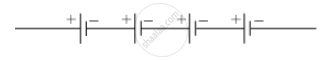SSC (English Medium) Class 9Maharashtra State Board
Share

# Electric Cells Having 2 V Potential Difference Each Have Been Connected in the Form of a Battery. What Will Be the Total Potential Difference of the Battery in Both Cases ? - SSC (English Medium) Class 9 - Science and Technology

ConceptPotential Difference

#### Question

Electric cells having 2 V potential difference each have been connected in the form of a battery. What will be the total potential difference of the battery in both cases ?

(i)(ii)#### Solution

(i) Total potential difference = 2 + 2 + 2 = 6 V

(ii) Total potential difference = 2 + 2 + 2 + 2 = 8 V

Is there an error in this question or solution?

#### APPEARS IN

Balbharati Solution for Balbharati Class 8 Science - General Science (2018 to Current)
Chapter 4: Current Electricity and Magnetism
Exercise | Q: 4 | Page no. 27
Solution Electric Cells Having 2 V Potential Difference Each Have Been Connected in the Form of a Battery. What Will Be the Total Potential Difference of the Battery in Both Cases ? Concept: Potential Difference.
S